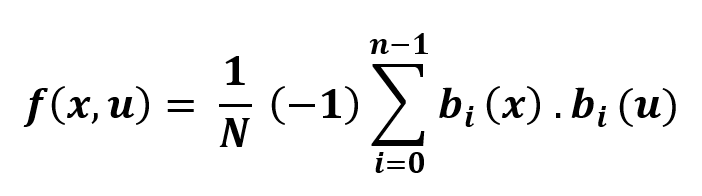GFG App
Open AppBrowser
Continue

# Python | Fast Walsh Hadamard Transform

Fast Walsh Hadamard Transform, is an Hadamard ordered efficient algorithm to compute the Walsh Hadamard transform (WHT). Normal WHT computation has N = 2m complexity but using FWHT reduces the computation to O(n2). The FWHT requires O(n logn) additions and subtraction operations. It is a divide and conquer algorithm which breaks down the WHT recursively.sympy.discrete.transforms.fwht( ) : It can perform Walsh Hadamard Transform (WHT). This method uses Hadamard sequence ordering.
Automatically the sequence is padded with zero to the right because the radix-2 FWHT requires the sample point number as a power of 2.

```Parameters :
-> seq : [iterable] sequence on which WHT is to be applied.

Returns :

Example #1 :

## Python3

 `# import sympy ` `from` `sympy ``import` `fwht`   `# sequence ` `seq ``=` `[``23``, ` `       ``56``, ` `       ``12``, ` `       ``555``]`   `# hwht` `transform ``=` `fwht(seq)` `print` `("Transform  : ", transform)`

Output :

`Transform  :  [646, -576, -488, 510]`

Example #2 :

## Python3

 `# import sympy ` `from` `sympy ``import` `fwht`   `# sequence ` `seq ``=` `[``15``, ``21``, ``13``, ``44``]`   `# hwht` `transform ``=` `fwht(seq)` `print` `("Transform  : ", transform)`

Output :

`Transform  :  [93, -37, -21, 25]`

My Personal Notes arrow_drop_up